﻿ Security Constrained Economic Environmental Dispatch through Fuzzy Based Gravitational Search Algorithm

### Security Constrained Economic Environmental Dispatch through Fuzzy Based Gravitational Search Algori...

P. K. Hota, N. C. SahuOPEN ACCESSPEER-REVIEWED

## Security Constrained Economic Environmental Dispatch through Fuzzy Based Gravitational Search Algorithm

P. K. Hota1,, N. C. Sahu2

1Department of Electrical Engineering Depart, Veer Surendra Sai University of Technology (VSSUT), Burla, India

2Department of Electrical Engineering, ITER, SOA University, Bhubaneswar, India

### Abstract

A robust and reliable algorithm called as fuzzy based gravitational search algorithm has been developed for solution of the security constrained economic and environmental load dispatch (EED) problems. A fuzzy maximizing decision approach using gravitational search algorithm (GSA) is applied to a six-unit thermal power test system to obtain the best compromising solution. The usefulness and quality of the proposed algorithm is demonstrated through its application to the test system in terms of convergence speed, solution time and minimum operating cost. The obtained results from the proposed algorithm confirm the effectiveness and potential of the promising proposed algorithm compared to conventional method, real coded genetic algorithm (GA), hybrid GA and PSO methods, performed in different central load dispatch centers to solve multi-objective generation dispatch problems.

### At a glance: Figures

12
Prev Next

• Hota, P. K., and N. C. Sahu. "Security Constrained Economic Environmental Dispatch through Fuzzy Based Gravitational Search Algorithm." American Journal of Electrical and Electronic Engineering 3.2 (2015): 44-50.
• Hota, P. K. , & Sahu, N. C. (2015). Security Constrained Economic Environmental Dispatch through Fuzzy Based Gravitational Search Algorithm. American Journal of Electrical and Electronic Engineering, 3(2), 44-50.
• Hota, P. K., and N. C. Sahu. "Security Constrained Economic Environmental Dispatch through Fuzzy Based Gravitational Search Algorithm." American Journal of Electrical and Electronic Engineering 3, no. 2 (2015): 44-50.

 Import into BibTeX Import into EndNote Import into RefMan Import into RefWorks

### 1. Introduction

The solution of nonlinear economic environmental load dispatch is to generate optimal amount of generating power from the fossil fuel based generating units in the system by minimizing the fuel cost and emission level simultaneously by satisfying all practical constraints. But, the objective of minimum cost of generation will not provide minimum pollution level and objective of minimum emission does not provide minimum cost of generation, which make both the objectives as non-commensurable and conflicting each other. Hence, they give rise to a set of optimal solutions instead of one optimal solution. The present situation demands to handle both objectives namely, economic dispatch and environmental emission as competing objectives and therefore, are to be solved simultaneously.

Nanda et al.  have treated Economic Emission Load Dispatch as a multiple, conflicting objective problem and solved using goal-programming techniques. Palanichamy et al.  demonstrated that reduction of harmful ecological effects caused by the emission of gaseous pollutants is possible only with a noticeable increase in the operating costs of the plants by the use of the familiar co-ordination equations approach. Nanda et al.  have also solved the Economic Emission Load Dispatch problem using a classical technique based on coordination equations to minimize the total cost of generation and pollution control simultaneously with varying degrees of compromise. Venkatesh et al.  showed that GA approach is the only viable solution to solve Economic load dispatch problem for the power system networks containing combined cycle cogeneration plants that have the cost characteristics which are not differentiable as it is not possible to solve such problems by conventional techniques. Kamaraj et al.  confirmed by solving economic dispatch problem that GA approach is efficient in handling multiple constraints. Baskar et al.  presented a two-phase Hybrid Genetic Algorithm based technique to solve emission and economic dispatch problems and demonstrated that their method is valid for real-time applications. Most of the approaches adopted above fail to address the nonlinear constrained EED problems satisfactory due to the complexity and non-monotonic nature of the problems. Sudhakaran et al.  have applied a refined genetic algorithm to combined economic and emission dispatch. In another paper, Sudhakaran et al. have solved the EED problem  by particle swarm optimization (PSO) to get better nondominated solutions within less execution time. However, the quality and diversity of the non-dominated solutions presented in  has not been measured and assessed quantitatively. The obvious weaknesses of this approach are that it is time-consuming and tends to find weakly non-dominated solutions. The classical techniques for solving EED problem need to introduce a compromise factor in order to decide the optimal solution and these results a complicated problem formulation. Further, these methods including GA and PSO techniques are not fast enough in terms of execution time. Moreover, they do not have a mechanism to show the vague or ‘fuzzy’ preference of the human decision-maker in obtaining a compromise solution in presence of such conflicting objectives.

On the other-hand, fuzzy systems provide tools for representing and manipulating inexact concepts and the ambiguity prevalent in human interpretation and thought processes. Further, fuzzy sets  can be applied for decision making in multiple objectives involving various constraints. Many interesting applications of fuzzy sets in the power field have been reported in the literature during last decade. Hota et al.  have described a simple and efficient technique based on fuzzy set theory for the EED problem. Further, they have developed an interactive fuzzy satisfying method  to solve multiobjective generation dispatch problem. The major advantage of fuzzy technique applied to this kind of problems lies in having a mechanism to show the vague/fuzzy preference of the human decision-maker in obtaining a compromising solution in the presence of conflicting objectives. However, the execution time of these fuzzy techniques appears not to be very promising for real time operation where the execution time is crucial. In recent times, practical power system optimization problems are being solved by various meta-heuristic algorithms successfully with quality solutions. However, there is no specific algorithm to achieve the best solution for all optimization problems. Some algorithms give a better solution for some particular problems than others. To investigate the gravitational search algorithm (GSA)  in solving the EED problem is the subject of this paper. GSA has been successfully applied to economic load dispatch (ELD) problem by Swain et al. . Further, Dugman et al  have also solved the ELD problem with valve point effects which provides the quality optimal generation dispatch solution with less execution time. However, in none of the application of GSA to EED problem, the security constraint has been considered.

In this paper, a fuzzy maximizing decision approach using gravitational search algorithm has been proposed to obtain the best compromising solution of the economic environmental dispatch problem considering security constraints. The obtained compromising solution permits the system operator to decide on different preferences for the objectives according to system operating conditions, thus resulting more flexible operation of generating units with improved man machine interface. The solutions so obtained by the proposed method show its robustness and superiority when compared with classical method, GA and PSO methods.

### 2. Problem Formulation

2.1. Economic Dispatch

The ELD problem may be expressed by minimizing the fuel cost of generating units under some constraints. The fuel cost curve is approximated as a quadratic function of the active power output from the generating units. Mathematically, the ELD problem can be defined as the following optimization problem.

Minimize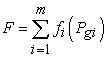,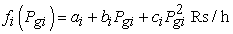(1)

where,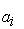,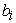and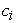are fuel cost coefficients of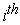generating unit;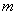is the total number of committed online generators; F is the total fuel cost; fi(Pgi) is the fuel cost ofgenerating unit which is a function of its real power generation Pgi.

Subject to

Power balance constraints or demand constraints: The total generation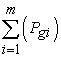should be equal to the total system demand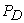plus the transmission network loss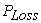.That is represented as,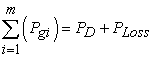(2)

The transmission losses must be taken into account in order to achieve true economic dispatch. To calculate the transmission losses, B-coefficients method and Newton-Raphson load flow solution are used in this paper. The real power loss PLoss in transmission lines can be calculated from Newton-Raphson load flow solution, which has equality constraints on real and reactive power at each bus as follows: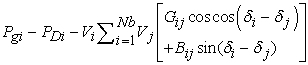(3)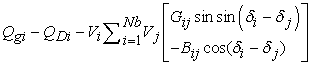(4)

where, i = 1, 2, …, Nb; Nb is the number of buses; Qgi is the reactive power generated at the ith bus; PDi and QDi are the real and reactive load power at ith bus, respectively; Gij and Bij are the transfer conductance and susceptance between bus i and bus j, respectively; Vi and Vj are voltage magnitudes at bus i and bus j, respectively; δi and δj are voltage angles at bus i and bus j, respectively.

The load flow solution by Newton-Raphson method is obtained as the real power output of one generator called slack generator, is left to cover the real power loss and satisfy the equality constraint in (2). It also gives all bus voltage magnitudes and angles. Then, the real power loss in transmission line can be calculated as: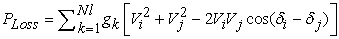(5)

where, Nl is the number of transmission lines and gk is the conductance of kth line that connects bus i to bus j.

The generator constraints: The generation real power of each unit should be between its minimum and maximum limits. That is, the following inequality constraint for each generator should be satisfied.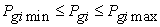(6)

where,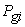is the real output ofgenerator and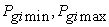are its minimum and maximum real power output ofgenerator, respectively.

Security constraints: It is quite essential that the operator must dispatch the online generating units such that the apparent power flow through the transmission line Si is restricted by its upper limits to ensure secured operation as described below.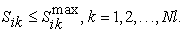(7)

It is to be noted that the kth transmission line flow connecting bus i and bus j can be calculated as: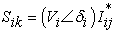where,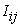is the current flow from bus i to bus j and can be calculated as: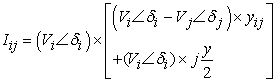(8)

where, yij is the line admittance and y is the shunt susceptance of the line.

2.2. Environmental Dispatch

The objective of emission dispatch is to minimize the total environmental degradation or the total pollutant emission due to the burning of fuels for production of power to meet the load demand. In the present work, however, SOx, NOx, CO2 and particulates, are treated together as a single pollution criterion. The amount of pollutants from a fossil based generating units depend on the amount of real power generated by that unit. Mathematically, the emission dispatch problem can be defined as the following optimization problem.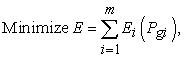(9)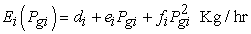(10)

where,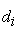,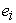and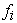are the pollution coefficients of thegenerating unit subjected to demand constraint and generating capacity constraints, as stated in eqn.2 and eqn.3; E is the total emission release;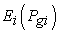is the emission release ofgenerating in Kg/hr.

2.3. Economic Environmental Dispatch

The non linear constrained multi-objective optimization problem can be converted to a single objective optimization problem (EED) as follows: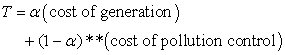(11)

where, T is total cost in Rs/hr;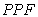is price penalty factor in Rs/kg and 0 < α< 1 is a compromise factor which is primarily a function of the preference of the decision maker. The total cost can be calculated as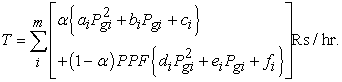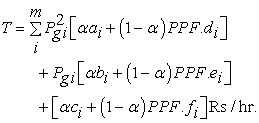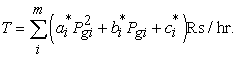(12)

where,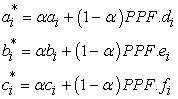The power system emission limit is expressed as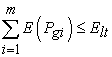(13)

where,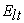is the total emission limit over the operating zones.

Therefore, the transformed single objective optimization problem can be described as: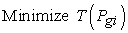(14)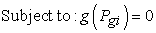(15)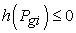(16)

where, g is the equality constraint representing the power balance equation, while h is the inequality constraint representing the generation capacity constraint.

### 3. Proposed Solution Methodology

3.1. Gravitational Search Algorithm

The gravitational search algorithm (GSA), is one of the newest metaheuristic search algorithm developed by Rashedi et al. in 2009 . GSA is followed by the physical law of gravity and the law of motion. The gravitational force between two particles is directly proportional to the product of their masses and inversely proportional to the square of the distance between them. In the proposed algorithm, agents are considered as objects and their performance is measured by their masses.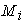is the mass of the of the object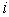,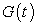is the gravitational constant at time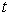, The gravitational constant, G, is initialized at the beginning and will be decreased with the time to control the search accuracy. In other words, G is function of the initial value (G0) and time (t):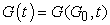(17)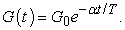(18)

The masses of the agents are calculated using fitness evaluation. A heavier mass means a more efficient agent. This means that better agents have higher attractions and moves more slowly. Supposing the equality of the gravitational and inertia mass, the values of masses are calculated using the map of fitness.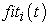represents the fitness value of the agentat time, and the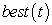and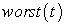in the population, respectively indicate the strongest and the weakness agent according to their fitness value.

For a minimization problem: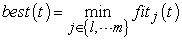(19)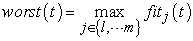(20)

For a Maximization problem: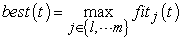(21)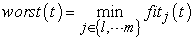(22)

The GSA consists of following steps such as i) Initialization, ii) Fitness evaluation (objective function), iii) Agent force calculation, iv) Evaluation of acceleration of an agent, v) Update the agents’ position and velocity and vi) Stopping criterion.

3.2. Best Compromising Solution

The fuzzy set theory has been implemented to derive efficiently a solution from the set of non-dominated solutions. The fuzzy decision making function is represented by the membership function to replace each variable as a precise value. Figure 1. depicts the membership function μi for the fuzzy variable signifying total fuel cost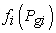. The decision maker is fully satisfied with the cost if μi =1, and not satisfied at all if μi = 0. Therefore, the value of membership function indicates the adaptability of the economy index. Due to the imprecise nature of decision maker’s judgment, theobjective function of a solution in the non-dominated setis represented by a membership function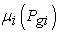defined as: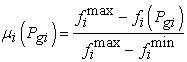(23)

where,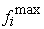and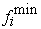are the maximum and minimum values ofobjective, respectively.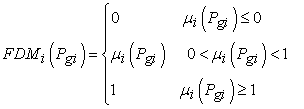(24)

For each non-dominated solution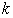, the normalized membership function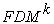is defined as: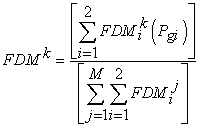(25)

The best compromising solution of EED problem is the one having the maximum value ofas fuzzy decision making function. Where, M is the total number of non dominated solutions. Then, all the solutions are arranged in descending order according to their membership function values which will guide the decision maker with a priority list of non-dominated solutions in view of the current operating conditions.

3.3. Computational Procedure of Proposed Fuzzy Based GSA Technique for EED

The computational procedure of the proposed fuzzy based GSA technique for the EED problem can be described as follows.

Step-1: Initialize Input parameters of the system.

Step-2: Initialize randomly the agents of the population. These agents must be feasible candidate solutions that satisfy the given constraints.

Step-3: Let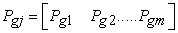be the trial vector denoting the particles of population to be evolved. The elements of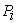are the real power outputs of the committed m generating units subjected to their respective capacity constraints in (3). To meet exactly the load demand in (2), a dependent unit is randomly selected from among the committed m units. Let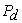be the power output of the dependent unit (slack generator), thenis calculated as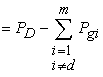,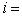1,2,….

Step-4: If the equation (2) is satisfied, go to step-6, otherwise go to step-2.

Step-5: Calculate the fitness value of each agent.

Step-6: Update gravitational constant G(t), best(t) and worst(t) and update the mass of the object Mi(t).

Step-7: Calculate Force in different directions.

Step-8: Calculate acceleration and velocity of each agent.

Step-9: Update the position of each agent.

Step-10: Repeat Step-5 to Step-9 until the stop criterion is satisfied.

Step-11: The agent that generates the latest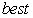(t) is the optimal value.

The flow chart of the overall computational procedure for EED using Fuzzy based GSA algorithm is shown in Figure 2.

### 4. Simulation Results

The proposed fuzzy set based GSA methodology is applied to the 6-generator system. The input data of the test system is adopted from  and system load demands are taken as 500 MW, 700MW and 900MW for comparison point of view.

The following key parameters are selected such as: M = 100, G0 = 120, α = 8,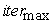(T) =50, etc.

#### Table 3. Economic Environmental dispatch (EED) results

For the load demand of 700MW, the EED problem is solved separately by proposed fuzzy based GSA and the results are compared with the conventional method, real coded GA, hybrid GA and PSO method in Table 1 and Table 2, respectively. The results proved that the proposed method is capable of providing non-dominated quality solutions within less execution time. It is evident from Table 3 that the solution obtained by the proposed method has minimum fuel cost, minimum emission and minimum loss.

Table 4 shows the optimal power output of generators for the EED problem. After getting many optimal solutions with varying degree of compromise with a load demand of 700 MW, the fuzzy set theory has been implemented to derive efficiently the best compromising solution in presence of conflicting objectives for the decision makers. The best compromising solution (10th one) is obtained with maximum FDM value as 0.1191 out of eleven non-dominated solutions as shown in Table 5.

#### Table 5. Non Dominated Solutions of Two Objectives with Fuzzy Decision Making Function

Figure 4. Convergence characteristics of proposed method with PD= 900 MW (EED problem)

#### Table 6. Generation Schedules corresponding to Non Dominated Solutions

Table 6 shows the best generation schedules for all non-dominated solutions. The best generation schedule corresponding to best compromising solution is highlighted in Table 6. The convergence characteristics of a six-unit system obtained by the proposed method for the load demand of 500 MW and 900 MW are shown in Figure 3 and Figure 4, respectively. The required number of iteration are found to be 28 and 32, respectively. Further, using the load flow program, the security constraints are checked and all the transmission lines are found to carry the real power in MW less than or equal to their corresponding maximum real power limits.

### 5. Conclusion

The proposed fuzzy based GSA technique has been implemented in the security constrained economic environmental load dispatch problems which, appears to be a simple and efficient optimization method. The obtained compromising solution permits the system operator to decide on different preferences for the objectives according to system operating conditions, thus resulting more flexible operation of generating units with improved man machine interface. The comparison of results for the test cases clearly identifies the superiority of the proposed method and is capable of obtaining reliable and efficient solutions in a short CPU time. Further, other practical objective functions such as reliability, etc. can also easily be incorporated in the proposed algorithm.

### Acknowledgements

The support from the Electrical Engineering Department of Veer Surendra Sai University of Technology, Burla, India, extended to the second author for the work reported in this paper is gratefully acknowledged.

### References

  Nanda J, Kothari D P, Linga Murthy K S, “Economic emission load dispatch through Goal Programming Techniques”, IEEE Trans. On Energy Conversion, 1988; Vol. l3, No 1, pp. 26-32.In article  Palanichamy C, Srikrishna K, “Economic thermal power dispatch with emission constraint”, Journal of the Institution of Engineers (India), pt. EL, 1991, Vol. 72, pp. 11-18.In article  Nanda J, Lakshman H, Kothari M L, “Economic emission load dispatch with line flow constraints using a Classical Technique”, IEE proceedings–Generation, Transmission and Distribution, January, 1994, Vol.141, No.1, pp. 1-10.In article  Venkatesh P, Kannan P S, Sudhakaran M, “Application of computational intelligence to economic load dispatch”, Journal of the Institution of Engineers (India), pt. EL, 2000, Vol. 81, pp. 39-43.In article  Kamaraj N, Sivakamasundari C, Kannan P S, “Economic dispatch with Line flow and emission constraints using genetic algorithm”, Journal of the Institution of Engineers (India), pt. EL, 2001, Vol. 82, pp. 195-199.In article  Baskar S, Subbaraj P, Rao M V C, “Hybrid genetic algorithm solution to emission and economic dispatch problems”, Journal of the Institution of Engineers (India), pt. EL, March, 2002, Vol. 82, pp. 343-249.In article  Sudhakaran M, Slochanal S M R, Sreeran R, Sekhar N C, “Application of refined genetic algorithm to combined economic and emission dispatch”, Journal of the Institution of Engineers (India), pt. EL, September, 2004, Vol. 85, pp. 115-119.In article  Sudhakaran M, Sivakumar G, Vengatachalapathy P, Latchumi K, “Particle swarm optimization for economic and emission dispatch problems”, Journal of the Institution of Engineers (India), pt. EL, 2007, Vol. 88, pp. 39-45.In article  Zimmermann H J, “Fuzzy Set Theory and Its Applications”, Kluwer-Nijhoff Publishing, 1985.In article  Hota P K, Chakrabarti R, Chattopadhyay P K, “A fuzzy-set based optimization technique for economic emission load dispatch”, Journal of the Institution of Engineers (India), pt. EL, November, 1999, Vol. 80, pp. 99-103.In article  Hota P K, Chakrabarti R, Chattopadhyay P K, “Economic emission load dispatch through an interactive fuzzy satisfying method”, Electric Power Systems Research, 2000, Vol. 54, No.3, pp. 151-157.In article  Rashedi E, Nezamabadi-pour H, Saryazdi S, “GSA: A Gravitational Search Algorithm”, Information Science, 2009, Vol. 179, pp. 2232-2248.In article  Swain R K, Sahu N C, Hota P K, “Gravitational Search Algorithm for Optimal Economic Dispatch”, Procedia Technology, Science Direct, Elsevier, ICCCS-2012, pp. 1-9.In article  Dugman S, Guvenc U, Yorukeren N, “Gravitational Search Algorithm for Economic Dispatch with Valve Point Effects”, International Review of Electrical Engineering, 2010, Vol. 5, No. 6, pp. 2890-2895.In article